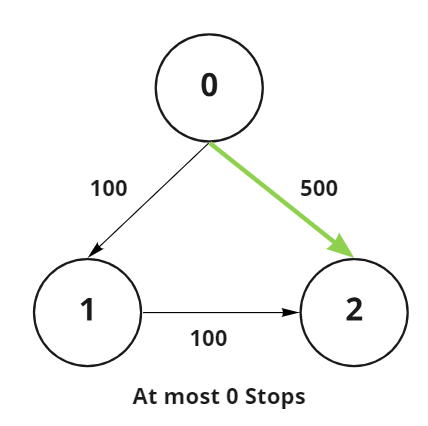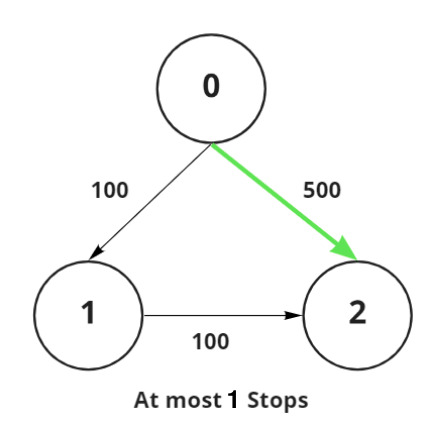Related Articles

# Maximum cost path from source node to destination node via at most K intermediate nodes

• Last Updated : 24 Mar, 2021

Given a directed weighted graph consisting of N vertices and an array Edges[][], with each row representing two vertices connected by an edge and the weight of that edge, the task is to find the path with the maximum sum of weights from a given source vertex src to a given destination vertex dst, made up of at most K intermediate vertices. If no such path exists, then print -1.

Examples:

Input: N = 3, Edges[][] = {{0, 1, 100}, {1, 2, 100}, {0, 2, 500}}, src = 0, dst = 2, K = 0
Output: 500
Explanation:Path 0 → 2: The path with maximum weight and at most 0 intermediate nodes is of weight 500.

Input: N = 3, Edges[][] = {{0, 1, 100}, {1, 2, 100}, {0, 2, 500}}, src = 0, dst = 2, K = 0
Output: 500
Explanation:Path 0 → 2: The path with maximum weight and at most 1 intermediate node is of weight 500.

Approach: The given problem can be solved by using BFS(Breadth-First Search) Traversal. Follow the steps below to solve the problem:

• Initialize the variable, say ans, to store the maximum distance between the source and the destination node having at most K intermediates nodes.
• Initialize an adjacency list of the graph using the edges.
• Initialize an empty queue and push the source vertex into it. Initialize a variable, say lvl, to store the number of nodes present in between src and dst.
• While the queue is not empty and lvl is less than K + 2 perform the following steps:
• Store the size of the queue in a variable, say S.
• Iterate over the range [1, S] and perform the following steps:
• Pop the front element of the queue and store it in a variable, say T.
• If T is the dst vertex, then update the value of ans as the maximum of ans and the current distance T.second.
• Traverse through all the neighbors of the current popped node and check if the distance of its neighbor is greater than the current distance or not. If found to be true, then push it in the queue and update its distance.
• Increase the value of lvl by 1.
• After completing the above steps, print the value of ans as the resultant maximum distance.

Below is the implementation of the above approach:

## C++

 `// C++ program for the above approach` `#include ``using` `namespace` `std;` `// Function to find the longest distance``// from source to destination with at``// most K intermediate nodes``int` `findShortestPath(``    ``int` `n, vector >& edges,``    ``int` `src, ``int` `dst, ``int` `K)``{``    ``// Initialize the adjacency list``    ``vector > > adjlist(``        ``n, vector >());` `    ``// Initialize a queue to perform BFS``    ``queue > q;` `    ``unordered_map<``int``, ``int``> mp;` `    ``// Store the maximum distance of``    ``// every node from source vertex``    ``int` `ans = INT_MIN;` `    ``// Initialize adjacency list``    ``for` `(``int` `i = 0; i < edges.size(); i++) {` `        ``auto` `edge = edges[i];` `        ``adjlist[edge].push_back(``            ``make_pair(edge, edge));``    ``}` `    ``// Push the first element into queue``    ``q.push({ src, 0 });` `    ``int` `level = 0;` `    ``// Iterate until the queue becomes empty``    ``// and the number of nodes between src``    ``// and dst vertex is at most to K``    ``while` `(!q.empty() && level < K + 2) {` `        ``// Current size of the queue``        ``int` `sz = q.size();` `        ``for` `(``int` `i = 0; i < sz; i++) {` `            ``// Extract the front``            ``// element of the queue``            ``auto` `pr = q.front();` `            ``// Pop the front element``            ``// of the queue``            ``q.pop();` `            ``// If the dst vertex is reached``            ``if` `(pr.first == dst)``                ``ans = max(ans, pr.second);` `            ``// Traverse the adjacent nodes``            ``for` `(``auto` `pr2 : adjlist[pr.first]) {` `                ``// If the distance is greater``                ``// than the current distance``                ``if` `(mp.find(pr2.first)``                        ``== mp.end()``                    ``|| mp[pr2.first]``                           ``> pr.second``                                 ``+ pr2.second) {` `                    ``// Push it into the queue``                    ``q.push({ pr2.first,``                             ``pr.second``                                 ``+ pr2.second });``                    ``mp[pr2.first] = pr.second``                                    ``+ pr2.second;``                ``}``            ``}``        ``}` `        ``// Increment the level by 1``        ``level++;``    ``}` `    ``// Finally, return the maximum distance``    ``return` `ans != INT_MIN ? ans : -1;``}` `// Driver Code``int` `main()``{``    ``int` `n = 3, src = 0, dst = 2, k = 1;``    ``vector > edges``        ``= { { 0, 1, 100 },``            ``{ 1, 2, 100 },``            ``{ 0, 2, 500 } };` `    ``cout << findShortestPath(n, edges,``                             ``src, dst, k);` `    ``return` `0;``}`

## Python3

 `# Python3 program for the above approach``from` `collections ``import` `deque` `# Function to find the longest distance``# from source to destination with at``# most K intermediate nodes``def` `findShortestPath(n, edges, src, dst, K):``    ` `    ``# Initialize the adjacency list``    ``adjlist ``=` `[[] ``for` `i ``in` `range``(n)]``    ` `    ``# Initialize a queue to perform BFS``    ``q ``=` `deque()` `    ``mp ``=` `{}` `    ``# Store the maximum distance of``    ``# every node from source vertex``    ``ans ``=` `-``10``*``*``9` `    ``# Initialize adjacency list``    ``for` `i ``in` `range``(``len``(edges)):``        ``edge ``=` `edges[i]``        ``adjlist[edge[``0``]].append([edge[``1``],``                                 ``edge[``2``]])` `    ``# Push the first element into queue``    ``q.append([src, ``0``])` `    ``level ``=` `0` `    ``# Iterate until the queue becomes empty``    ``# and the number of nodes between src``    ``# and dst vertex is at most to K``    ``while` `(``len``(q) > ``0` `and` `level < K ``+` `2``):` `        ``# Current size of the queue``        ``sz ``=` `len``(q)` `        ``for` `i ``in` `range``(sz):``            ` `            ``# Extract the front``            ``# element of the queue``            ``pr ``=` `q.popleft()``            ` `            ``# Pop the front element``            ``# of the queue``            ``# q.pop()` `            ``# If the dst vertex is reached``            ``if` `(pr[``0``] ``=``=` `dst):``                ``ans ``=` `max``(ans, pr[``1``])` `            ``# Traverse the adjacent nodes``            ``for` `pr2 ``in` `adjlist[pr[``0``]]:``                ` `                ``# If the distance is greater``                ``# than the current distance``                ``if` `((pr2[``0``] ``not` `in` `mp) ``or``                  ``mp[pr2[``0``]] > pr[``1``] ``+` `pr2[``1``]):``                      ` `                    ``# Push it into the queue``                    ``q.append([pr2[``0``], pr[``1``] ``+` `pr2[``1``]])``                    ``mp[pr2[``0``]] ``=` `pr[``1``] ``+` `pr2[``1``]` `        ``# Increment the level by 1``        ``level ``+``=` `1` `    ``# Finally, return the maximum distance``    ``return` `ans ``if` `ans !``=` `-``10``*``*``9` `else` `-``1` `# Driver Code``if` `__name__ ``=``=` `'__main__'``:``    ` `    ``n, src, dst, k ``=` `3``, ``0``, ``2``, ``1` `    ``edges``=` `[ [ ``0``, ``1``, ``100` `],``             ``[ ``1``, ``2``, ``100` `],``             ``[ ``0``, ``2``, ``500` `] ]` `    ``print``(findShortestPath(n, edges,src, dst, k))` `# This code is contributed by mohit kumar 29`
Output:
`500`

Time Complexity: O(N + E)
Auxiliary Space: O(N)

Attention reader! Don’t stop learning now. Get hold of all the important DSA concepts with the DSA Self Paced Course at a student-friendly price and become industry ready.  To complete your preparation from learning a language to DS Algo and many more,  please refer Complete Interview Preparation Course.

In case you wish to attend live classes with experts, please refer DSA Live Classes for Working Professionals and Competitive Programming Live for Students.

My Personal Notes arrow_drop_up